# Lab 18.3 Multilevel Collections

 Team-Fly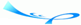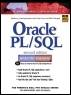Oracle® PL/SQL® Interactive Workbook, Second EditionBy Benjamin Rosenzweig, Elena Silvestrova Table of Contents Chapter 18.  Collections

Lab Objective

After this Lab, you will be able to:

• Use Multilevel Collections

So far you have seen various examples of collections with the element type based on a scalar type, such as NUMBER and VARCHAR2. In Oracle 9i, PL/SQL provides you with the ability to create collections whose element type is based on a collection type. Such collections are called multilevel collections. You will recall that multilevel collections is a new feature and is not supported by Oracle versions prior to 9i.

Consider a varrray of varrays shown in Figure 18.3.

##### Figure 18.3. A Varray of Varrays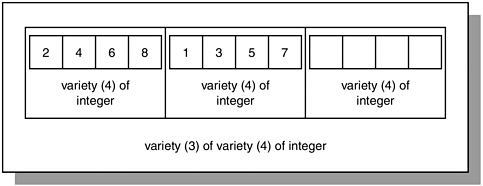Figure 18.3 shows a varray of varrays or nested varray. A varray of varrays consists of three elements, where each individual element is a varray consisting of four integer numbers. As a result, in order to reference an individual element of a varray of varrays, you use the following notation:

` varray_name(subscript of the outer varray)(subscript of  the inner varray) `

For example, the varray(1)(3) in Figure 18.3 equals 6; similarly, varray(2)(1) equals 1.

Consider an example based on Figure 18.3.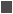FOR EXAMPLE

` DECLARE     TYPE varray_type1 IS VARRAY(4) OF INTEGER;     TYPE varray_type2 IS VARRAY(3) OF varray_type1;     varray1 varray_type1 := varray_type1(2, 4, 6, 8);     varray2 varray_type2 := varray_type2(varray1);  BEGIN     DBMS_OUTPUT.PUT_LINE ('Varray of integers');     FOR i IN 1..4 LOOP        DBMS_OUTPUT.PUT_LINE ('varray1('||i||'): ' ||           varray1(i));     END LOOP;     varray2.EXTEND;     varray2(2) := varray_type1(1, 3, 5, 7);     DBMS_OUTPUT.PUT_LINE (chr(10)||        'Varray of varrays of integers');     FOR i IN 1..2 LOOP        FOR j IN 1..4 LOOP           DBMS_OUTPUT.PUT_LINE              ('varray2('||i||')('||j||'): '||varray2(i)(j));        END LOOP;     END LOOP;  END; `

In this declaration portion of the example, you define two varray types. The first type, varray_type1, is based on the INTEGER datatype and can contain up to four elements. The second type, varray_type2, is based on the varray_type1 and can contain up to six elements. Next, you declare two varrays based on the types just described. The first varray, varray1, is declared as varray_type1 and initialized so that its four elements are populated with the first four even numbers. The second varray, varray2, is declared as varray_type2, so that each individual element is a varray consisting of four integer numbers, and initialized so that it first varray element is populated.

In the executable portion of the example, you display the values of the varray1 on the screen. Next, you extend the upper bound of the varray2 by one, and populate its second element as follows:

` varray2(2) := varray_type1(1, 3, 5, 7); `

Notice that you are using a constructor corresponding to the varray_type1 because each element of the varray2 is based on the varray1 collection. In other words, the same result could be achieved via the following two statements:

` varray1 := varray_type1(1, 3, 5, 7);  varray2 := varray_type2(varray1); `

Once the second element of the varray2 is populated, you display results on the screen via nested numeric FOR loops.

This example produces the following output:

` Varray of integers  varray1(1): 2  varray1(2): 4  varray1(3): 6  varray1(4): 8  Varray of varrays of integers  varray2(1)(1): 2  varray2(1)(2): 4  varray2(1)(3): 6  varray2(1)(4): 8  varray2(2)(1): 1  varray2(2)(2): 3  varray2(2)(3): 5  varray2(2)(4): 7  PL/SQL procedure successfully completed. `

#### Lab 18.3 Exercises

##### 18.3.1 Use Multilevel Collections

In this exercise, you will learn more about multilevel collections.

Create the following PL/SQL script:

` -- ch18_4a.sql, version 1.0  SET SERVEROUTPUT ON  DECLARE     TYPE table_type1 IS TABLE OF integer INDEX BY     BINARY_INTEGER;     TYPE table_type2 IS TABLE OF table_type1 INDEX BY     BINARY_INTEGER;     table_tab1 table_type1;     table_tab2 table_type2;  BEGIN     FOR i IN 1..2 LOOP        FOR j IN 1..3 LOOP           IF i = 1 THEN              table_tab1(j) := j;           ELSE              table_tab1(j) := 4 - j;           END IF;           table_tab2(i)(j) := table_tab1(j);           DBMS_OUTPUT.PUT_LINE ('table_tab2('||              i||')('||j||'): '||table_tab2(i)(j));        END LOOP;     END LOOP;  END; `

Execute the script, and then answer the following questions:

 a) Execute the script ch18_4a.sql and explain the output produced. b) Modify the script so that instead of using multilevel index-by tables it uses a nested table of index-by tables. c) Modify the script so that instead of using multilevel index-by tables it uses a nested table of varrays.

#### Lab 18.3 Exercise Answers

This section gives you some suggested answers to the questions in Lab 18.3, with discussion related to how those answers resulted. The most important thing to realize is whether your answer works. You should figure out the implications of the answers here and what the effects are from any different answers you may come up with.

##### 18.3.1 Answers

 a) Execute the script ch18_4a.sql and explain the output produced. A1: Answer: Your output should look similar to the following:` table_tab2(1)(1): 1 table_tab2(1)(2): 2 table_tab2(1)(3): 3 table_tab2(2)(1): 3 table_tab2(2)(2): 2 table_tab2(2)(3): 1 PL/SQL procedure successfully completed. `The script ch18_4a.sql uses multilevel index-by tables or an index-by table of index-by tables. The declaration portion of the script defines a multilevel index-by table table_tab2. Each row of this table is an index-by table consisting of three rows. The executable portion of the script populates the multilevel table via nested numeric FOR loops. In the first iteration of the outer loop, the inner loop populates the index-by table table_tab1 with values 1, 2, 3, and the first row of the multilevel table table_tab2. In the second iteration of the outer loop, the inner loop populates the index-by table table_tab1 with values 3, 2, 1, and the second row of the multilevel table table_tab2. b) Modify the script so that instead of using multilevel index-by tables it uses a nested table of index-by tables. A2: Answer: Your script should look similar to the following script. Changes are shown in bold letters.` -- ch18_4b.sql, version 2.0 SET SERVEROUTPUT ON DECLARE TYPE table_type1 IS TABLE OF integer INDEX BY BINARY_INTEGER; TYPE table_type2 IS TABLE OF table_type1; table_tab1 table_type1; table_tab2 table_type2 := table_type2(); BEGIN FOR i IN 1..2 LOOP table_tab2.EXTEND; FOR j IN 1..3 LOOP IF i = 1 THEN table_tab1(j) := j; ELSE table_tab1(j) := 4 - j; END IF; table_tab2(i)(j) := table_tab1(j); DBMS_OUTPUT.PUT_LINE ('table_tab2('|| i||')('||j||'): '||table_tab2(i)(j)); END LOOP; END LOOP; END; `In this version of the script, the table_type2 is declared as a nested table of index-by tables. Next, table_tab2 is initialized prior to its use, and its size is extended before a new element is assigned a value. c) Modify the script so that instead of using multilevel index-by tables it uses a nested table of varrays. A3: Answer: Your script should look similar to the following script. Changes are shown in bold letters.` -- ch18_4c.sql, version 3.0 SET SERVEROUTPUT ON DECLARE TYPE table_type1 IS VARRAY(3) OF integer; TYPE table_type2 IS TABLE OF table_type1; table_tab1 table_type1 := table_type1(); table_tab2 table_type2 := table_type2(table_tab1); BEGIN FOR i IN 1..2 LOOP table_tab2.EXTEND; table_tab2(i) := table_type1(); FOR j IN 1..3 LOOP IF i = 1 THEN table_tab1.EXTEND; table_tab1(j) := j; ELSE table_tab1(j) := 4 - j; END IF; table_tab2(i).EXTEND; table_tab2(i)(j):= table_tab1(j); DBMS_OUTPUT.PUT_LINE ('table_tab2('|| i||')('||j||'): '||table_tab2(i)(j)); END LOOP; END LOOP; END; `In this declaration section of the script, the table_type1 is defined as a varray with three integer elements, and the table_type2 is declared as a nested table of varrays. Next, table_tab1 and table_tab2 are initialized prior to their uses.In the executable portion of the script, the size of the table_tab2 is incremented via the EXTEND method and its individual elements are initialized as follows:` table_tab2(i) := table_type1(); `Notice that that each element is initialized via the constructor associated with the varray type table_type1. Furthermore, in order to populate a nested table, a new varray element must be added to the each nested table element as shown:` table_tab2(i).EXTEND; `In other words, for the first iteration of the outer loop, there are three varray elements added to the first element of the nested table. Without this statement, the script causes the following error:` DECLARE * ERROR at line 1: ORA-06533: Subscript beyond count ORA-06512: at line 20 `When run, this script produces output identical to the original example:` table_tab2(1)(1): 1 table_tab2(1)(2): 2 table_tab2(1)(3): 3 table_tab2(2)(1): 3 table_tab2(2)(2): 2 table_tab2(2)(3): 1 PL/SQL procedure successfully completed. `

##### Answers

4)

Based on the preceding script, what is the value of the variable VAR1?

1. _____ There is no value because the script generates an error.

2. _____ 2

3. _____ 6

A1:

Questions

Answers

Comments

4)

C

Consider the statement

` varray2(2)(3) `

In this statement you are referencing the second element of varray2 and third element of varray1. Each element of varray2 is a varray of three integers defined as varray1. Recall the following declaration statement:

` varray2 varray_type2 :=     varray_type2(varray1, varray_type1     (4, 5, 6)); `

where varray_type1(4, 5, 6) is the second element of the varray2. Notice that the third element of varray1 is 6. As a result, the variable var1 is assigned a value of 6.

5)

The statement varray2(3).EXTEND

1. _____ adds a third element to the third element of VARRAY2.

2. _____ adds a second element to the third element of VARRAY2.

3. _____ causes a 'Subscript beyond count' error.

A2:

Questions

Answers

Comments

5)

B

You will recall that the PL/SQL block contains the following statements:

` varray2.EXTEND;  varray2(3) := varray_type1(0);  varray2(3).EXTEND; `

The first statement increases the size of the varray2. In other words, this statement adds the third element to the collection. The second statement initializes the third element of the varray2 via constructor associated with the varray type varray_type1. This is done because each element of the varray2 is a varray of three integers. This adds one element to the varray1. The third statement increases the size of the varray1 by adding a placeholder for the second element. In other words, it adds the second element to the third element of varray2.

#### Lab 18.3 Self-Review Questions

In order to test your progress, you should be able to answer the following questions.

Answers appear in Appendix A, Section 18.3.

 1) Multilevel collections are not supported by Oracle 8i. _____ True_____ False 2) A varray of varrays has an upper bound _____ that is fixed and cannot be extended to all._____ that can be extended to its maximum size._____ that can be extended without any limits. 3) There is no need to initialize a nested table of index-by tables prior to its use. _____ True_____ False

Consider the following script for the next two questions:

` DECLARE     TYPE varray_type1 IS VARRAY(3) OF INTEGER;     TYPE varray_type2 IS VARRAY(10) OF varray_type1;     varray1 varray_type1 := varray_type1(1, 2, 3);     varray2 varray_type2 := varray_type2(varray1,     varray_type1(4, 5, 6));     var1 INTEGER;  BEGIN     var1 := varray2(2)(3);     varray2.EXTEND;     varray2(3) := varray_type1(0);     varray2(3).EXTEND;  END; `

 4) Based on the preceding script, what is the value of the variable VAR1? _____ There is no value because the script generates an error._____ 2_____ 6 5) The statement varray2(3).EXTEND _____ adds a third element to the third element of VARRAY2._____ adds a second element to the third element of VARRAY2._____ causes a 'Subscript beyond count' error.

 Team-FlyTop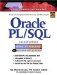Oracle PL/SQL Interactive Workbook (2nd Edition)
ISBN: 0130473200
EAN: 2147483647
Year: 2002
Pages: 146

Similar book on Amazon

flylib.com © 2008-2017.
If you may any questions please contact us: flylib@qtcs.net